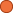## ← Output Solution - Intro to Computer Science

• 4 Followers
• 33 Lines

### Get Embed Code x Embed video Use the following code to embed this video. See our usage guide for more details on embedding. Paste this in your document somewhere (closest to the closing body tag is preferable): ```<script type="text/javascript" src='https://amara.org/embedder-iframe'></script> ``` Paste this inside your HTML body, where you want to include the widget: ```<div class="amara-embed" data-url="http://www.youtube.com/watch?v=Je2vSCmDP-0" data-team="udacity"></div> ``` 9 Languages

•English [en] original
•Spanish [es]
•Italian [it] incomplete
•Japanese [ja]
•Portuguese, Brazilian [pt-br]
•Russian [ru]
•Ukrainian [uk]
•Chinese, Simplified [zh-cn] incomplete
•Chinese, Traditional [zh-tw]

Showing Revision 7 created 05/25/2016 by Udacity Robot.

1. So this is a little bit trickier.
2. To answer this one we have to look at the code after the procedure,
3. and remember we said the procedure is replacing these four lines.
4. After the procedure what we do is print the value of "url."
5. So that means we definitely need at least "url" as our output
6. and all of the choices here included "url."
7. But that's not all we do, so we have to look at the second line as well.
8. This uses both "page" and "end_quote."
9. The value of "page" here is the same value of "page" before the procedure
10. So we don't actually need to return the value of "page."
11. It doesn't need to be an output from the procedure.
12. We already know that.
13. We knew that before we called the procedure.
14. The procedure doesn't change the value of "page."
15. No sense making it an output.
16. So this answer doesn't make sense. We don't need "page."
17. It does make sense to make "end_quote" one of the outputs.
18. The reason we want "end_quote" as an output is
19. we need to know where the end of the quote was
20. to advance the page, so the next time we look for the next target,
21. we don't find the same one that we just found.
22. So we actually need this, so this is the best answer to the question,
23. is that we need two outputs, we need both the url, and the value of "end_quote."
24. The fourth possibility could work.
25. If we return both the url and the value of "start_link,"
26. We could figure out a way to advance the page to not find the same quote,
27. but we'd basically have to redo all this code.
28. We need to look for the next quote, starting from "start_link."
29. We need to look for the closing quote for that.
30. Basically we'd need to compute the value of "end_quote" again.
31. So it's a lot more useful to return the value of "end_quote,"
32. than to return the value of "start_link."
33. That's why the third choice is the best choice.